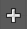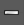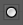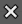# Doc:2.6/Manual/Materials/Nodes/Types/Vector

< Doc:2.6‎ | Manual‎ | Materials‎ | Nodes‎ | Types

# Material Vector Nodes

Vector nodes manipulate information about how light interacts with the material, multiplying vector sets, and other wonderful things that normal humans barely comprehend (except math geniuses, who may not be considered «normal»). Even if you aren't a math wiz, you'll find these nodes to be very useful.

Vectors, in general, are two or three element values, for example, surface normals are vectors. Vectors are also important for calculating shading.

## Normal Node

The Normal node generates a normal vector and a dot product. Click and Drag on the sphere to set the direction of the normal.

This node can be used to input a new normal vector into the mix. For example, use this node as an input to a Color Mix node. Use an Image input as the other input to the Mixer. The resulting colorized output can be easily varied by moving the light source (click and dragging the sphere).

The (face) normal is the direction of the face in relation to the camera. You can use it to do the following:

• Use this node to create a fixed direction -> output Normal.
• Calcuate the Dot-Product with the Normal-Input. The Dot-Product is a scalar value (a number).
• If two normals are pointing in the same direction the Dot-Product is 1.
• If they are perpendicular the Dot-Product is zero (0).
• If they are antiparallel (facing directly away from each other) the Dot-Product is -1. And you never thought you would use the Vector Calculus class you took in college - shame on you!

So now we can do all sorts of things that depends on the viewing angle (like electron scanning microscope effect). And the best thing about it is that you can manipulate the direction interactively.

 One caveat The normal is evaluated per face, not per pixel. So you need enough faces, or else you don't get a smooth result

### Inputs

Normal
3D-direction of the face in relation to the camera. The value can be provided by another node or set manually.

### Outputs

Normal
Fixed 3D-direction, combined by the node.
Dot
Scalar value (a number), combined by the node.

### Controls

Interactive node preview
Allows click and drag on the sphere in node center to set the direction of the normal.

## Mapping Node

Essentially mapping node allows the user to modify a mapping of system of 3D-coordinates. Typically used for modifying texture coordinates.

Mapping can be rotated and clamped if desired.

### Inputs

Vector
The input vector (3D-direction in relation to the camera) of some the coordinates' mapping. The value can be provided by another node or set manually.

### Outputs

Vector
The output vector, combined by the node.

### Controls

The controls of the node have been ordered in X, Y, Z order. If you want to use the clamping options, try enabling Min and Max.

Vector type selector
Type of vector that the mapping transforms.
Texture
Transform a texture by inverse mapping the texture coordinates.
Point
Transform a point.
Vector
Transform a direction vector.
Normal
Transform a normal vector with unit length.

Location
Transform position vector.
Rotation
Transform rotation vector.
Scale
Transform scale vector.

Min
Minimum clipping value.
Max
Maximum clipping value.

## Vector Curves

The Vector Curves node maps an input vector x, y, and z components to a diagonal curve. Use this node to remap a vector value using curve controls.

Click and drag along the curve to create a control point and to change the curve's shape. Use the X to delete the selected (white) point.

### Inputs

Fac
Factor. The degree of node's influence in node tree. The value can be provided by another node or set manually.
Vector
The input vector (3D-direction in relation to the camera). The value can be provided by another node or set manually.

### Outputs

Vector
The output vector, combined by the node.

### Controls

Channel selector
Allows to select appropriate curve channel.
X
Curve of X-direction.
Y
Curve of Y-direction.
Z
Curve of Z-direction.Zoom in curve.Zoom out curve.

Reset View
Resets view of the cuve.
Vector Handle
Vector type of curve point's handle.
Auto Handle
Automatic type of curve point's handle.
Extend Horizontal
Extends the curve horizontal.
Extend Extrapolated
Extends the curve extrapolated.
Reset Curve
Resets the curve in default (removes all added curve's points).Clipping options display of the curve.Deletes points of the curve.